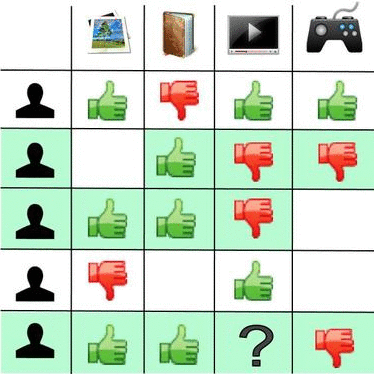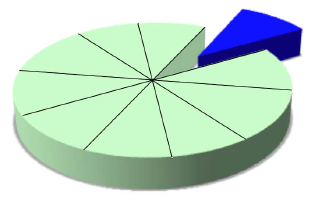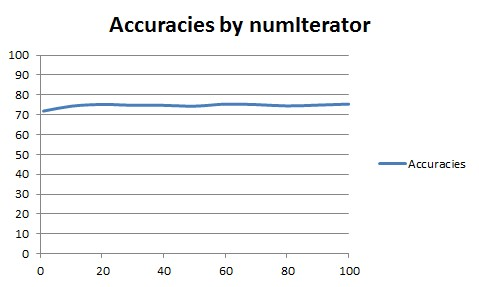# Machine Learning - (Overfitting|Overtraining|Robust|Generalization) (Underfitting)

A learning algorithm is said to overfit if it is:

• more accurate in fitting known data (ie training data) (hindsight) but
• less accurate in predicting new data (ie test data) (foresight)

Ie the model do really wel on the training data but really bad on real data. If this case, we say that the model can't be generalized.

In statistics and machine learning, overfitting occurs when a statistical model describes random error or noise instead of the underlying relationship.

• “Overfitting” is when a classifier fits the training data too tightly.
• Such a classifier works well on the training data but not on independent test data.
• Overfitting is a general problem that plagues all machine learning methods.

Low error on training data and high error on test data

Overfitting occurs when a model begins to memorize training data rather than learning to generalize from trend.

The more difficult a criterion is to predict (i.e., the higher its uncertainty), the more noise exists in past information that need to be ignored. The problem is determining which part to ignore.

Overfitting generally occurs when a model is excessively complex, such as having too many parameters relative to the number of observations.

When your learner outputs a classifier that is 100% accurate on the training data but only 50% accurate on test data, when in fact it could have output one that is 75% accurate on both, it has overfit.

Pedro Domingos - A Few Useful Things to Know About Machine Learning, CACM 55(10), 2012

Overfitting is when you create a model which is predicting the noise in the data rather than the real signal.

## Graphic Representation

The ingredients of prediction error are actually:

• bias: the bias is how far off on the average the model is from the truth.
• and variance. The variance is how much that the estimate varies around its average.

Bias and variance together gives us prediction error.

### Model Complexity vs Prediction Error

where:

Model complexity decreases prediction error until a point (the bias trade-off) where we are adding just noise. The trainer error goes down as it has to, but the test error is starting to go up. That's over fitting.

We want to find the model complexity that gives the smallest test error.

See:

## Example

If the number of parameters is the same as or greater than the number of observations, a simple model or learning process can perfectly predict the training data simply by memorizing the training data in its entirety, but such a model will typically fail drastically when making predictions about new or unseen data, since the simple model has not learned to generalize at all.

It' easy to demonstrate “overfitting” with a numeric attribute. Example with the weather data set and the temperature numeric attribute

``````if temperature in (83, 64, 72, 81, 70, 68, 75, 69, 75) then 'Play'
else if temperature in (65, 71, 85, 80, 72) then 'Don''t Play'```
```

There is one condition by observation and therefore the rules fit to much the (training) data.

## How to

### Measure

How badly algorithms overfit can be judged in terms of the apparent performance improvement between training set(s) and test set(s) with the help of the following measures:

• standard errors of estimators where standard error is the standard deviation of the new estimates under resampling. (ie by getting new samples over and over again and by recomputing our estimate).

Ideally, in order to calculate them, we get a new test sample from the population and see how well our predictions do.

But it's very often not possible to have a new one then if:

• we have a large test set, we can resample
• or we don't have a large test set, we can adjust the training error to get the test error with the help of mathematical methods that are going to increase it by a factor that involves the amount of fitting that we've done to the data and the variance. These methods could be:

Difference between the fit on training data and test data measures the model’s ability to generalize.

A algorithm that get 100% accurate on the training set overfits dramatically.

### Avoid

An algorithm is less likely to overfit:

By using several algorithm in order to make the good decision is also a good solution to avoid over-fitting.

In order to avoid overfitting in a algorithm, it is necessary to use additional techniques

• on parameters:

## Model and Overfitting

boosting seems to not overfit (Why boosting does'nt overfit)

## Glossary

### Robust

A learning algorithm that can reduce the chance of fitting noise is called robust.

### Generalize

Is the model able to generalize ? Can the model deal with unseen data, or does it overfit the data?

Generalizing is finding pattern in order to not overfit.

See Evaluation

## Documentation / Reference

Discover More(Machine learning|Inverse problems) - Regularization

Regularization refers to a process of introducing additional information in order to: solve an ill-posed problem or to prevent overfitting. This information is usually of the form of a penalty...(Prediction|Recommender System) - Collaborative filtering

Collaborative filtering is a method of making automatic predictions (filtering) the interests of a user by collecting preferences or taste information from many users (collaborating). But in general,...(Statistics|Data Mining) - (K-Fold) Cross-validation (rotation estimation)

Cross-validation, sometimes called rotation estimation is a resampling validation technique for assessing how the results of a statistical analysis will generalize to an independent new data set. This...Data Mining - (Test|Expected|Generalization) Error

Test error is the prediction error that we incur on new data. The test error is actually how well we'll do on future data the model hasn't seen. The test error is the average error that results from using...Data Mining - (Boosting|Gradient Boosting|Boosting trees)

Boosting forces new classifiers to focus on the errors produced by earlier ones. boosting works by aggressively reducing the training error Gradient Boosting is an algorithm based on an ensemble of decision...Data Mining - (Dimension|Feature) (Reduction)

In machine learning and statistics, dimensionality reduction is the process of reducing the number of random variables (features) under consideration and can be divided into: feature selection (returns...Data Mining - (Function|Model)

The model is the function, equation, algorithm that predicts an outcome value from one of several predictors. During the training process, the models are build. A model uses a logic and one of several...Data Mining - Decision Tree (DT) Algorithm

Desicion Tree (DT) are supervised Classification algorithms. They are: easy to interpret (due to the tree structure) a boolean function (If each decision is binary ie false or true) Decision trees...Data Mining - Decision boundary Visualization

Classifiers create boundaries in instance space. Different classifiers have different biases. You can explore them by visualizing the classification boundaries. Logistic Regression method produces...Data Mining - Information Gain

Information theory was find by Claude_ShannonClaude Shannon. It has quantified entropy. This is key measure of information which is usually expressed by the average number of bits needed to store or communicate...